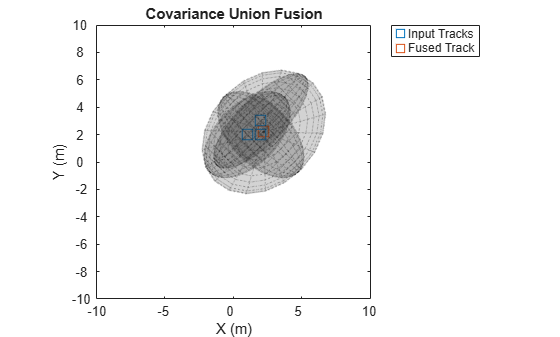# fusecovunion

Covariance fusion using covariance union

## Syntax

``[fusedState,fusedCov] = fusecovunion(trackState,trackCov)``

## Description

example

````[fusedState,fusedCov] = fusecovunion(trackState,trackCov)` fuses the track states in `trackState` and their corresponding covariance matrices `trackCov`. The function estimates the fused state and covariance in a way that maintains consistency. For more details, see Consistent Estimator.```

## Examples

collapse all

Define a state vector of tracks.

```x(:,1) = [1;2;0]; x(:,2) = [2;2;0]; x(:,3) = [2;3;0];```

Define the covariance matrices of the tracks.

```p(:,:,1) = [10 5 0; 5 10 0;0 0 1]; p(:,:,2) = [10 -5 0; -5 10 0;0 0 1]; p(:,:,3) = [12 9 0; 9 12 0;0 0 1];```

Estimate the fused state vector and its covariance.

`[fusedState,fusedCov] = fusecovunion(x,p);`

Use `trackPlotter` to plot the results.

```tPlotter = theaterPlot('XLim',[-10 10],'YLim',[-10 10],'ZLim',[-10 10]); tPlotter1 = trackPlotter(tPlotter, ... 'DisplayName','Input Tracks','MarkerEdgeColor',[0.000 0.447 0.741]); tPlotter2 = trackPlotter(tPlotter, ... 'DisplayName','Fused Track','MarkerEdgeColor',[0.850 0.325 0.098]); plotTrack(tPlotter1,x',p) plotTrack(tPlotter2, fusedState', fusedCov) title('Covariance Union Fusion')```## Input Arguments

collapse all

Track states, specified as an N-by-M matrix, where N is the dimension of the state and M is the number of tracks.

Data Types: `single` | `double`

Track covariance matrices, specified as an N-by-N-by-M array, where N is the dimension of the state and M is the number of tracks.

Data Types: `single` | `double`

## Output Arguments

collapse all

Fused state, returned as an N-by-1 vector, where N is the dimension of the state.

Fused covariance matrix, returned as an N-by-N matrix, where N is the dimension of the state.